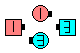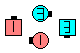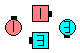Definitions of Square Dance Calls and Concepts

Index -->  Plus  |  A1  |  A2  |  C1  |  C2  |  C3A  |  C3B  |  C4  |  NOL  |
Definitions (Text Only) -->  Plus  |  A1  |  A2  |  C1  |  C2  |  C3A  |  C3B  |  C4  |  NOL  |
 Find call:

Single 1/4 Tag \$B\$+\$i!%(B

Scoot Back \$B\$r\$7\$F(B, Outsides \$B\$,(B handhold (\$B\$H\$C\$F\$\$\$k

Diamond \$B\$G=*\$o\$j\$^\$9!%(BScoot The Diamond\$B\$NA0(B after Scoot Back & Outsides 1/4 To Handhold Diamond Circulate\$B\$N8e(B (\$B=*\$o\$j(B)

Scoot The formation [NOL]: Scoot Back \$B\$r\$7\$F(B, Outsides (Scoot Back \$B\$N8e\$Kformation Circulate \$B\$r\$7\$^\$9!%(B

Scoot The Triangle [NOL]: Wave-Based Triangle \$B\$G(B Apex \$B\$,(B Base \$B\$NJ}\$r8~\$\$\$F\$\$\$k\$H\$3\$m\$+\$i!%(B Scoot Back \$B\$r\$7\$F(B, Apex \$B\$N?M\$,(B handhold (\$B\$H\$C\$F\$\$\$kScoot The Magic Diamond [NOL]: 1/4 Tag \$B\$+\$i!%(B Scoot Back \$B\$r\$7\$F(B, Outsides \$B\$,(B handhold (\$B\$H\$C\$F\$\$\$kMagic Diamond Circulate \$B\$r\$7\$^\$9!%(B Twin Diamonds \$B\$G=*\$o\$j\$^\$9!%(B

Scoot The Exchange The Diamond [NOL]: 1/4 Tag \$B\$+\$i!%(B Scoot Back \$B\$r\$7\$F(B, Outsides \$B\$,(B handhold (\$B\$H\$C\$F\$\$\$kExchange The Diamond \$B\$r\$7\$^\$9!%(B Twin Diamonds \$B\$G=*\$o\$j\$^\$9!%(B

Scoot Chain Thru The Diamond [NOL]: 1/4 Tag \$B\$+\$i!%(B Scoot Chain Thru \$B\$r\$7\$F(B, Outsides \$B\$,(B handhold (\$B\$H\$C\$F\$\$\$k \$B

• Interlocked Scoot The Diamond [C3A]:
Interlocked Scoot Back \$B\$r\$7\$F(B, Outsides \$B\$,(B handhold (\$B\$H\$C\$F\$\$\$k
• Scoot The Interlocked Diamond [NOL]:
1/4 Tag \$B\$+\$i!%(B Scoot Back \$B\$r\$7\$F(B, Outsides \$B\$,(B handhold (\$B\$H\$C\$F\$\$\$k
•CALLERLAB definition for Scoot The DiamondChoreography for Scoot The DiamondComments? Questions? Suggestions?